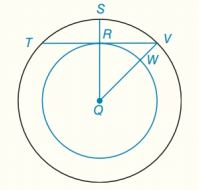Chapter 6.CT, Problem 8CT### Elementary Geometry for College St...

6th Edition
Daniel C. Alexander + 1 other
ISBN: 9781285195698

#### Solutions

Chapter
Section### Elementary Geometry for College St...

6th Edition
Daniel C. Alexander + 1 other
ISBN: 9781285195698
Textbook Problem
1 views

# a) Because point Q is their common center, these circles are known as                                                         _ circles.b) If R Q = 3 and Q V = 5 , find the length of chord T V ¯ which is also a tangent to the inner circle.                                   _To determine

(a)

To fill:

The blank in the statement “Because point Q is their common center, these circles are known as _ circles”.

Explanation

Definition used:

Concentric circles are coplanar circles that have a common center.

Given:

Q is the common center for two circles.

Approach:

Clearly, Q is the common center for two circles

To determine

(b)

To find:

The length of chord TV¯ which is tangent to the inner circle.

### Still sussing out bartleby?

Check out a sample textbook solution.

See a sample solution

#### The Solution to Your Study Problems

Bartleby provides explanations to thousands of textbook problems written by our experts, many with advanced degrees!

Get Started

#### In Problems 15-28, find the general solution to the given differential equation.

Mathematical Applications for the Management, Life, and Social Sciences

#### j × (−k) = i −i j + k −j − k

Study Guide for Stewart's Multivariable Calculus, 8th

#### For what values of p does the series converge?

Study Guide for Stewart's Single Variable Calculus: Early Transcendentals, 8th

#### Simplify. 25a2b(2a2ba+b2)

Mathematics For Machine Technology